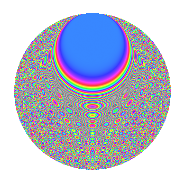# Properties

 Label 2100.2.bgLevel 2100 Weight 2 Character orbit bg Rep. character $$\chi_{2100}(851,\cdot)$$ Character field $$\Q(\zeta_{6})$$ Dimension 584 Sturm bound 960

# Related objects

## Defining parameters

 Level: $$N$$ $$=$$ $$2100 = 2^{2} \cdot 3 \cdot 5^{2} \cdot 7$$ Weight: $$k$$ $$=$$ $$2$$ Character orbit: $$[\chi]$$ $$=$$ 2100.bg (of order $$6$$ and degree $$2$$) Character conductor: $$\operatorname{cond}(\chi)$$ $$=$$ $$84$$ Character field: $$\Q(\zeta_{6})$$ Sturm bound: $$960$$

## Dimensions

The following table gives the dimensions of various subspaces of $$M_{2}(2100, [\chi])$$.

Total New Old
Modular forms 1008 632 376
Cusp forms 912 584 328
Eisenstein series 96 48 48

## Trace form

 $$584q + 2q^{4} - 16q^{6} + 2q^{9} + O(q^{10})$$ $$584q + 2q^{4} - 16q^{6} + 2q^{9} + 2q^{12} + 8q^{13} - 14q^{16} - 10q^{21} + 28q^{22} - 18q^{24} + 2q^{28} - 10q^{33} + 24q^{34} + 12q^{36} + 16q^{42} - 16q^{46} + 28q^{48} + 24q^{49} - 4q^{52} + 8q^{54} + 4q^{57} + 2q^{58} - 4q^{61} + 44q^{64} - 38q^{66} + 44q^{69} - 14q^{72} + 24q^{73} - 72q^{76} + 8q^{78} - 34q^{81} + 44q^{82} - 18q^{84} + 26q^{88} + 22q^{93} + 20q^{94} + 24q^{96} + 24q^{97} + O(q^{100})$$

## Decomposition of $$S_{2}^{\mathrm{new}}(2100, [\chi])$$ into newform subspaces

The newforms in this space have not yet been added to the LMFDB.

## Decomposition of $$S_{2}^{\mathrm{old}}(2100, [\chi])$$ into lower level spaces

$$S_{2}^{\mathrm{old}}(2100, [\chi]) \cong$$ $$S_{2}^{\mathrm{new}}(84, [\chi])$$$$^{\oplus 3}$$$$\oplus$$$$S_{2}^{\mathrm{new}}(420, [\chi])$$$$^{\oplus 2}$$

## Hecke characteristic polynomials

There are no characteristic polynomials of Hecke operators in the database